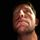Active IQ Unified Manager Discussions

## WFA Volume resize, TargetSize concats in place of sum4,209 Views

I would like summarize actual vol size of a volume with an added value

unfortunately that join rather summarize, I saw type is [capacity].

Is that a way to perform arithmetic operation in this field?

1 ACCEPTED SOLUTION4,200 Views

You can't do arithmetic addition in the field directly.  The + operator works as concatentation there.  You can utilize a function, though, to do the match for you.  I created this function:

{
num1 = (float)(num1);
num2 = (float)(num2);
return (num1 + num2);
}

It forces each number to a floating point number and them adds them together and returns the value.  In this case, you would put this in the TargetSize field:

Good luck!

Brian Jones

5 REPLIES 54,201 Views

You can't do arithmetic addition in the field directly.  The + operator works as concatentation there.  You can utilize a function, though, to do the match for you.  I created this function:

{
num1 = (float)(num1);
num2 = (float)(num2);
return (num1 + num2);
}

It forces each number to a floating point number and them adds them together and returns the value.  In this case, you would put this in the TargetSize field:

Good luck!

Brian Jones4,196 Views

Strange thing

this works:

(((long)createvolumebin.size_mb) + ((long)(\$volsizebin)))

this not:

(((long)createvolumebin.size_mb) + ((long)(\$volsizebin *1024)))2,698 Views

francoisbnc, what version of WFA were you using for (((long)createvolumebin.size_mb) + ((long)(\$volsizebin)))?

I'm using version 5 and getting illegal expression.4,191 Views

Anyway, it works well with your solution thanks2,700 Views

Brian, where did you add the function you created? Inside the PowerShell or Perl code for the 'resize volume' command?